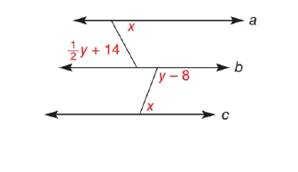Chapter 2.1, Problem 39EElementary Geometry For College St...

7th Edition
Alexander + 2 others
ISBN: 9781337614085

Solutions

Chapter
SectionElementary Geometry For College St...

7th Edition
Alexander + 2 others
ISBN: 9781337614085
Textbook Problem

For the figure shown, a ∥ b ∥ c , Find x and y.To determine

To find:

The values of x, and y.

Explanation

Given:

The given figure is,

abc.

Definition:

If there exists two parallel lines cut by a transversal then the alternate angles will be same.

The sum of angles that falls on the straight line is 180°.

Calculation:

Consider the figure,

Figure (1)

m1=x, m2=12y+14 , m3=y8 and m5=x

From figure (1) ab.

1 and 2 are alternate angles.

m1=m2x=12y+142x=y+28 ...(1)

From the figure (1), bc.

3 and 4 fall on the line b.

m3+m4=180°y8+m4=180°m4=180°y+8°m4=188°y

From the figure (1), bc

Still sussing out bartleby?

Check out a sample textbook solution.

See a sample solution

The Solution to Your Study Problems

Bartleby provides explanations to thousands of textbook problems written by our experts, many with advanced degrees!

Get Started

If f is even, then f is even.

Single Variable Calculus: Early Transcendentals, Volume I

Describe the set of points (x,y) such that x2+y2=9.

Finite Mathematics and Applied Calculus (MindTap Course List)

Find for x = 3t2 + 1, y = t6 + 6t5. t4 + 5t3 4t3 + 15t2

Study Guide for Stewart's Multivariable Calculus, 8th

True or False: The graph at the right is the graph of an even function.

Study Guide for Stewart's Single Variable Calculus: Early Transcendentals, 8th

In Exercises 14, find the distance between the two points. (2,3) and (1,7)

Finite Mathematics for the Managerial, Life, and Social Sciences